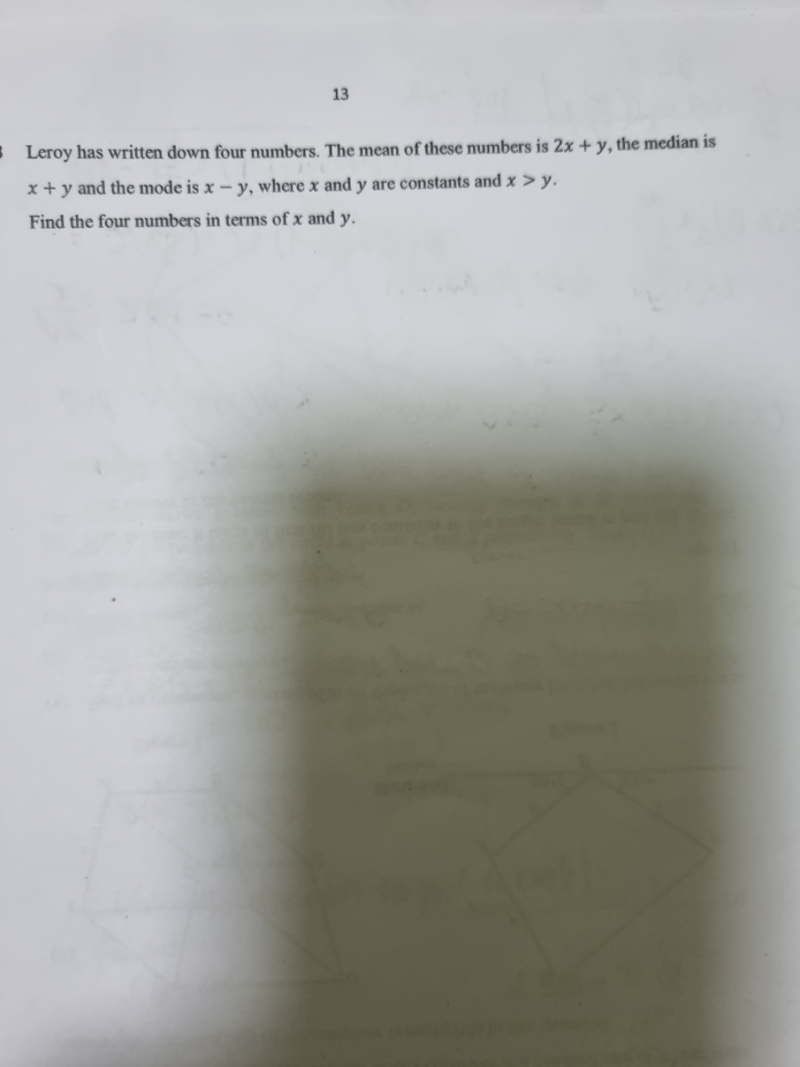# QuestionThank you

Hi,

For those questions that I have answered earlier, 3 of them you have not accepted.  If the answers do not match your answer, can you please furnish the answer key instead of leaving it in limbo?   I can explain if the answer key is wrong or if I am wrong, I can make the correction.

Solution for this question:

Mean = 2x + y => Total = 4 (2x + y) =  8x +4y

Median = x + y is one of the 4 numbers

Mode = x – y means at least 2 numbers are x-y

So 8x + 4y – (x+y) – 2(x-y) = 8x + 4y – x – y – 2x + 2y = 5x + 5y

Hence the 4 numbers are : 5x + 5y, x + y, x – y and  x-y